# Why does the internal energy depend on the temperature

### The internal energy U is a state functionWe will now investigate the consequences of the fact that dU is a total differential. First of all, we note that for a closed system of constant composition U is a function of volume and temperature. Basically U is a function of V, T and p; however, these quantities are not independent, but are linked to one another by the equation of state. If we give V and T, then p is also determined. For our purposes it is best to choose V and T as variables, but we could also choose p, T or p, V as independent state variables.

Now let us assume that both V and T change in infinitesimal steps and get for the infinitesimal change of the internal energy: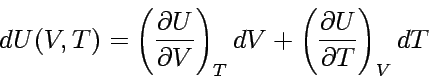In a closed system with constant composition, every infinitesimal change in internal energy is proportional to the respective changes in volume and temperature. The proportionality factors are the partial derivatives according to the state variables, here V and T.

In general, a partial derivative is the slope of the graph of a quantity of interest as a function of one Variables with which this quantity is functionally related; all other variables are kept constant. In many cases, this slope can be interpreted physically directly. In this case it is the differential quotient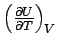the heat capacity: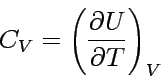We see this when we remember that dU = dq - pdV, because it follows: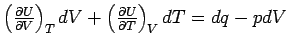or after forming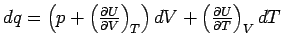and at constant volume (dV = 0) it becomes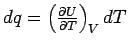which is nothing more than our relationship between temperature and heat, namely heat capacity.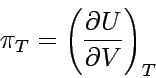The coefficient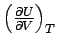, also has an important thermodynamic meaning: It is a measure of the change in the internal energy of a substance when its volume changes at constant temperature. We denote it with πTbecause it has the dimension of a print.

So that is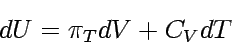If during the isothermal expansion of a substance (dV> 0) its internal energy increases (dU> 0) - this is the case when forces of attraction act between particles - the graph of internal energy as a function of volume has a positive slope, π appliesT > 0. If there is no interaction between the particles, the internal energy does not depend on the mutual distance between the particles and therefore also does not depend on the volume of the sample; for an ideal gas is therefore πT = 0. This last equation, i.e. the statement that volume and internal energy are independent of one another, is a definition of the ideal gas; it is equivalent to the equation of state pV = nRT.

Since for an ideal gas U = 3/2 RT applies, i.e. U does not depend on V, must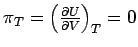be. For CV, m of an ideal gas we get: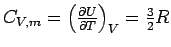.

Partial derivatives have many useful properties. By using the partial differential quotient appropriately, one can often transform unknown quantities into known, interpretable or measurable ones. For example, how does the internal energy change depending on the temperature at constant pressure of the system?

From the equation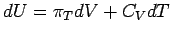follows: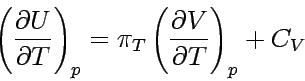The differential quotient on the right side of the equation is the slope of the graph of the function V (T) at constant pressure. This value can be found tabulated as the coefficient of thermal expansion α of a substance, it is defined as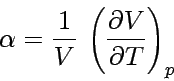By substituting the general equation for defining α in the relationship for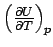you get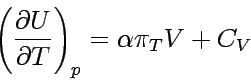.

This is a general equation for closed systems of constant composition. It describes the dependence of the internal energy on the temperature at constant pressure as a function of C.V (can be measured experimentally), α (obtainable by a further measurement) and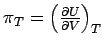. For an ideal gas is πT = 0 and thus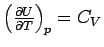(ideal gas).

Note that the equation only applies in the special case of the ideal gas, while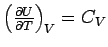must always apply as the definition of heat capacity!

By the end of this chapter we will deal with an ideal gas whose heat capacity does not depend on temperature. By restricting to an ideal gas, πT = 0, we get dU = CVdT. As for one adiabatic Expansion / compression dq = 0, it follows that dU = dw and therefore: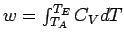Now we add the condition that the heat capacity should be independent of temperature; so it turns out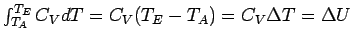The work involved in the adiabatic change in volume of an ideal gas is then proportional to the temperature difference between the initial and final state.

In general, one can deduce from the last equation that for w <0 (i.e. the system does work) ΔT <0 applies. This is not surprising: since no heat can get into the system, its internal energy has to decrease when the system is doing work. The internal energy of an ideal gas is not influenced by changes in volume; therefore, as the internal energy decreases, the temperature must decrease.The data protection declaration of the TU Braunschweig applies to this website with the exception of Sections VI, VII and VIII.